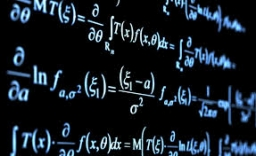# Derivative problem

The sum of two numbers is 12. Find these numbers if:
a) The sum of their third powers is minimal.
b) The product of one with the cube of the other is maximal.
c) Both are positive and the product of one with the other power of the other is maximal.

Correct result:

a1 =  6
b1 =  6
a2 =  3
b2 =  9
a3 =  4
b3 =  8

#### Solution:

$a+b=12 \ \\ y=min(a^3+b^3) \ \\ y=min(a^3+(12-a)^3) \ \\ \ \\ f'=3a^2 -3(12-a)^2 \ \\ f'=0 \ \\ \ \\ \ \\ 3 \cdot \ x^2 -3 \cdot \ (12-x)^2=0 \ \\ \ \\ 72x=432 \ \\ \ \\ x=6 \ \\ \ \\ a_{1}=6$
$b_{1}=12-a_{1}=12-6=6$
$f_{2}=a \cdot \ b^3 \ \\ f_{2}=a \cdot \ (12-a)^3 \ \\ f_{2}'(a)=d/da(a (12 - a)^3)=-4 (a - 3) (12 - a)^2 \ \\ \ \\ f_{2}'(a)=0 \ \\ \ \\ m_{1}=3 \ \\ m_{2}=12 \ \\ m_{3}=12 \ \\ \ \\ f_{21}=m_{1} \cdot \ (12-m_{1})^3=3 \cdot \ (12-3)^3=2187 \ \\ f_{22}=m_{2} \cdot \ (12-m_{2})^3=12 \cdot \ (12-12)^3=0 \ \\ \ \\ \ \\ a_{2}=m_{1}=3$
$b_{2}=12-a_{2}=12-3=9$

Our quadratic equation calculator calculates it.

$b_{3}=12-a_{3}=12-4=8$We would be pleased if you find an error in the word problem, spelling mistakes, or inaccuracies and send it to us. Thank you!Tips to related online calculators

## Next similar math problems:

• Secret treasureScouts have a tent in the shape of a regular quadrilateral pyramid with a side of the base 4 m and a height of 3 m. Determine the radius r (and height h) of the container so that they can hide the largest possible treasure.
• Two pipesHow long will the pool be filled with a double supply pipe if it takes the pool to fill the first pipe by 4 hours longer and the second pipe 9 hours longer than both pipes open at the same time?
• PoolIf water flows into the pool by two inlets, fill the whole for 19 hours. The first inlet filled pool 5 hour longer than the second. How long pool take to fill with two inlets separately?
• Right triangleLegs of the right triangle are in the ratio a:b = 2:8. The hypotenuse has a length of 87 cm. Calculate the perimeter and area of the triangle.
• Two carsTwo cars started against each other at the same time to journey long 346 km. The first car went 53 km/h and the second 53 km/h. What distance will be between these cars 20 minutes before meet?
• Body diagonalThe cuboid has a volume of 32 cm3. Its side surface area is double as one of the square bases. What is the length of the body diagonal?
• Pyramid cutWe cut the regular square pyramid with a parallel plane to the two parts (see figure). The volume of the smaller pyramid is 20% of the volume of the original one. The bottom of the base of the smaller pyramid has a content of 10 cm2. Find the area of the
• Rectangular cuboidThe rectangular cuboid has a surface area 5334 cm2, its dimensions are in the ratio 2:4:5. Find the volume of this rectangular cuboid.
• Two trucksTwo trucks left cities A and B against each other and met after an hour. The first car came to B 27 minutes later than the second car to A. Calculate the car speed if the distance between cities A, B is 90 km.
• SquareIf the length of the sides of the square we decrease by 25% decrease the content area of 28 cm2. Determine the side length of the original square.
• Four integersFnd four consecutive integers so that the product of the first two is 70 times smaller than the product of the next two.
• Digit sumThe digit sum of the two-digit number is nine. When we turn figures and multiply by the original two-digit number, we get the number 2430. What is the original two-digit number?
• Minimum surfaceFind the length, breadth, and height of the cuboid shaped box with a minimum surface area, into which 50 cuboid shaped blocks, each with length, breadth and height equal to 4 cm, 3 cm and 2 cm respectively can be packed.
• Cuboid and ratioFind the dimensions of a cuboid having a volume of 810 cm3 if the lengths of its edges coming from the same vertex are in ratio 2: 3: 5
• A rectangle 2A rectangle has a diagonal length of 74cm. Its side lengths are in ratio 5:3. Find its side lengths.
• 1 page1 page is torn from the book. The sum of the page numbers of all the remaining pages is 15,000. What numbers did the pages have on the page that was torn from the book?
• Cube in a sphereThe cube is inscribed in a sphere with volume 7253 cm3. Determine the length of the edges of a cube.